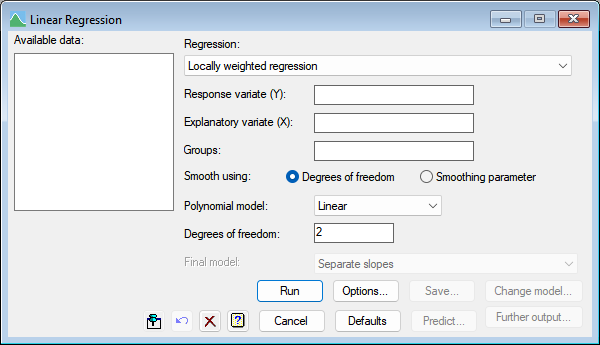1. Home
2. Locally Weighted Regression

# Locally Weighted Regression

Select menu: Stats | Regression Analysis | Linear Models

Locally weighted regression (a loess curve) downdown list option fits a model based on one x-variate. The assumption in the modelling is that the locally, around any point, the regression surface can be approximated by a function from a particular class: polynomials of order 1 (linear) or 2 (quadratic).## Response variate

Specifies the name of the response (or y-) variate.

## Explanatory variate

Specifies the name of the explanatory (or x-) variate.

## Smooth using

Controls whether approximate degrees of freedom or a smoothing parameter are to be supplied.

## Degrees of freedom

Specifies the degrees of freedom from which Genstat searches for the appropriate span to generate a smoothing model with the required degrees of freedom.

## Smoothing parameter

Specifies the span to indicate what proportion of points are used to fit the regression model at each position.

## Polynomial model

Specifies the polynomial model to be used. You can choose either Linear or Quadratic.

## Groups

If the data values are classified into groups you can supply a factor defining the different categories. When a grouping factor is supplied a series of models are fitted.

The first model fitted (Common curve) is a locally weighted regression, ignoring the groups. The second model (Parallel curves) is extended to include a different constant (or intercept) for each group, giving a set of parallel curves one for each group. The third model (Separate slopes) fits a common locally weighted regression component to all groups, but allows each group to have a separate constant and linear slope term. The final model (Separate curves) has different constants, slopes and locally weighted regressions for each group. The Final model list below the Groups field lets you select between the types of regression model that you want to fit.

## Final model

This option is disabled until Run has been used, so that it fits all models and then lets you select the most appropriate model after reviewing the fits. If the analysis shows that different intercepts or slopes or loess terms are needed, you can use this option to select the final model and re-run the analysis to remove the interaction between the locally weighted regression and the groups factor. Similarly, if different intercepts or slopes are not needed this option can be used to fit just a common locally weighted regression.

## Action IconsPin Controls whether to keep the dialog open when you click Run. When the pin is downthe dialog will remain open, otherwise when the pin is upthe dialog will close.Restore Restore names into edit fields and default settings.Clear Clear all fields and list boxes.Help Open the Help topic for this dialog.